# Analysis

The underlying focus of mathematical analysis is the study of functions. An enormous number of the mathematical models that have been developed over the years to study the economic, physical, natural and social sciences describe the behaviour of real-world systems using functions to represent the objects being modelled, and the ways in which these objects evolve and interact is represented by equations involving functions, such as ordinary and partial differential equations.

Some of these (especially nonlinear equations) are too complex to be solved using current technology, and we do not know, for instance, whether initially smooth solutions to the equations of fluid flow can develop turbulent behaviour. The members of the analysis group study equations involving functions, and in some cases, study the properties of functions and ways in which they can be represented, in order to improve our capacity to tackle the equations.

### Professor Jonathan Bennett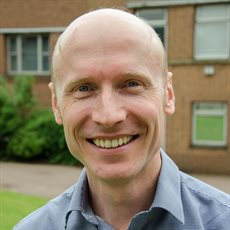Professor of Mathematical Analysis

Professor Bennett's interests lie in multivariable Euclidean harmonic analysis and its interactions with problems in geometric analysis and combinatorics. Recently he has been investigating the scope of heat-flow methods and induction-on-scales arguments in the analysis of geometric inequalities arising in the restriction theory for the Fourier transform.

### Dr Hong Duong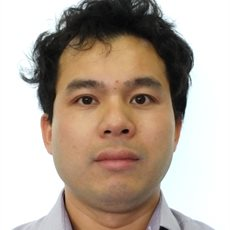Associate Professor

Dr Duong's research interests lie in the intersections of analysis and applied probability. Most of his research is inspired from applications in molecular dynamics, material sciences and biological systems.

### Dr Michael Dymond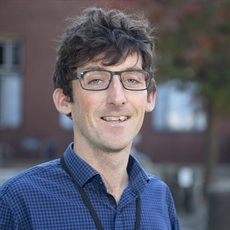Assistant Professor

Dr Dymond's research interests lie in the intersection of functional analysis, geometric measure theory and discrete mathematics. He studies Lipschitz mappings in a range of different settings and their relation to exceptional sets, such as porous and sigma porous sets.

### Professor Chris Good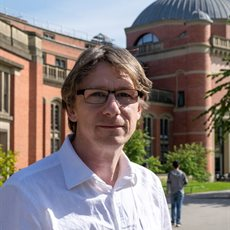Professor of Mathematics

Professor Good's research considers: characterisations of maps with the shadowing property; dynamical systems with a topological rather than metric phase space; the Auslander-Yorke dichotomy, equicontinuity, transitivity and sensitivity; countable dynamical systems; induced dynamics on hyperspaces and function spaces; coarse graining of complex systems.

### Dr Susana Gutierrez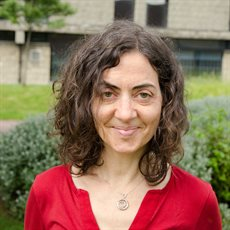Senior Lecturer

Dr Gutierrez's research concerns the rigourous analytical study of qualitative and quantitative properties of partial differential equations motivated by models of well-known physical processes. A recurring theme in her research is the application of techniques and perspectives from euclidean harmonic analysis and dispersive PDEs.

### Dr Jingyu Huang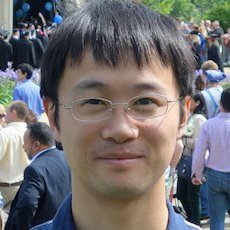Lecturer

Dr Huang's primary areas of research involve stochastic analysis and stochastic partial differential equations.

### Dr Marina Iliopoulou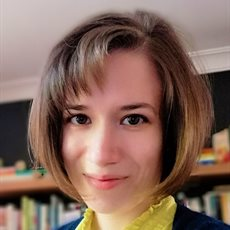Associate Professor

Dr Iliopoulou's interests are in harmonic analysis, incidence geometry, geometric measure theory, and additive combinatorics.

### Dr Sabrina Kombrink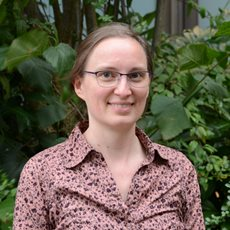Assistant Professor of Mathematics
Senior Tutor (J-BJI)

Sabrina is interested in geometrically characterising highly irregular objects as well as in the question which geometric features one can hear when listening to the sound of a fractal drum. Her research lies in the intersection of analysis, geometry and stochastics.

### Professor Olga Maleva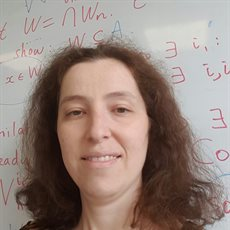Professor of Mathematics

Professor Maleva's research is on differentiability of Lipschitz maps and the geometry of exceptional sets, particularly with relation to the converse of Rademacher's theorem. She works on establishing finer measure-theoretic regularity properties of universal differentiability sets and sets on which Lipschitz maps behave in the worst possible way, as well as typical Lipschitz functions.

### Dr John Meyer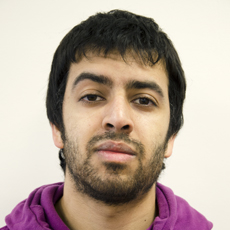Associate Professor in Applied Mathematics

Dr Meyer's research is in the field of analysis of nonlinear differential equations and is interested in the following areas: reaction-diffusion theory, maximum principles, quasi-linear parabolic partial differential equations, chemical reaction modelling.

### Dr Andrew Morris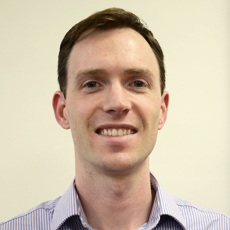Associate Professor in Mathematical Analysis

Dr Morris develops techniques in harmonic analysis, functional calculus and geometric measure theory for application to PDEs on Riemannian manifolds and rough domains. This includes elliptic systems with rough coefficients, local T(b) techniques, first-order methods, holomorphic functional calculus, singular integral theory, Hardy spaces, boundary value problems and uniform rectifiability.

### Dr Tony Samuel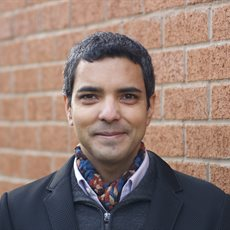Associate Professor of Mathematics
Chair of Information Technology and Digital Delivery

Tony's mathematical research interests lie in aperiodic order (mathematical quasicrystals), Diophantine approximations, dynamical systems, fractal geometry, and numeration systems. More recently he has become interested in developing interactive educational materials using RStudio and the Python library Manim.

### Dr Yuzhao Wang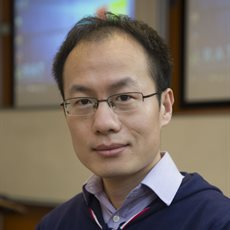Associate Professor

Dr Wang's primary research area is mathematical analysis of nonlinear dispersive PDEs, with tools from harmonic analysis, probability theory, and dynamical systems. In particular, he is interested in Strichartz estimates and its applications to dispersive nonlinear PDEs, probabilistic aspects of nonlinear dispersive PDEs, and normal form method applied to nonlinear dispersive PDEs.

## Research and Teaching Fellows

### Dr Engin Başakoğlu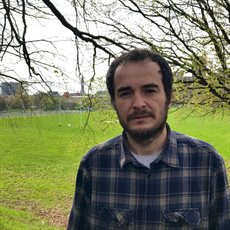Research Fellow

Dr Basakoglu’s research area is the analysis of nonlinear dispersive PDEs. More precisely, his primary research interest is to analyze the behavior of solutions of initial value/initial-boundary value problems of dispersive PDEs. Also, he is interested in the dynamics of dispersive PDEs perturbed by singular stochastic forcing.

### Dr Itamar Oliveira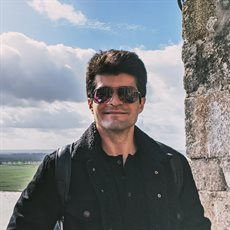Research Fellow

Dr Oliveira's research is broadly in linear and multilinear harmonic analysis. He is mainly interested in understanding the influence of underlying curvature features on the behaviour of integral operators, such as in the Fourier restriction program and in the study of classical modulation-invariant singular integral operators.

## PhD Students

Note that not all PhD researchers have requested profiles.